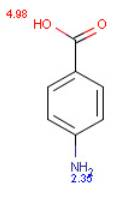# pKa Plugin

## 1. Introduction

Chemical properties of molecules depend largely on whether they are ionized or not. Most organic molecules are capable of gaining and/or losing a proton in aqueous solutions. Proton transfer most frequently occurs between water and any ionizable atom of the organic molecule. The molecule's response to protonation or deprotonation depends significantly on the site that was disturbed by proton transfer. Partial charge distribution in the molecule also varies with protonation of the acid/base active sites. Since partial charge distribution is very sensitive to the protonation-deprotonation process (both near and far from the disturbed site), it can be used to determine the pKa of a molecule. Our pKa prediction program is based on the calculation of partial charge of atoms in the molecule.

## 2. Definition of Acid Dissociation Constant Ka

Acidic and basic molecules are ionized in aqueous solution. Acidic or basic character is assigned to the molecule according to Brönsted's rule. The ratio of the ionized and neutral forms depends on the pH, the temperature and the ion activity of the bulk phase. The ionization constant Ka is obtained from the activity ratio of conjugated base and conjugated acid multiplied with proton activity.## 3. Definition of pKa

pKa is obtained from the ionization constant of a molecule using the following definition.When the pH of the solution is equal with pKa, the concentrations of dissociated and undissociated species are equal.

## 4. Multiprotic Molecules

When a molecule contains more than one ionizable atom, it is called a multiprotic compound. For these types of molecules we need to distinguish between micro and macro acidic dissociation constants. The micro acidic dissociation constant is obtained from the equilibrium concentration of the conjugated acid-base pairs. The macro acidic dissociation constant is obtained from the global mass and charge conservation law. When a molecule has N ionizable sites, the total number of micro species in the solution is 2N.

To further understand the difference between micro and macro constants we consider ionization equilibrium of a triprotic acid AH3. Referring to the different deprotonation sites of the AH3 molecule we introduce the upper indexes of the protons.The ionization process of the AH1H2H3 molecule in aqueous solution is described with 12 equilibrium reactions. Micro species and their charge are summarized in Table 1.

## 5. Ionization Steps of the AH3 MoleculeTable 1. Microspecies and Their Charge
 Microspecies charge AH1H2H3 0 AH1H2 , AH1H3 , AH2H3 -1 AH1 , AH2 , AH3 -2 A -3

The AH3 molecule has three macro acidic constants since it is triprotic acid. Macro acidic constants K1, K2, and K3 are obtained from the concentration of the micro species.And the three macro pKa values of the AH3 molecule that would be obtained with routine laboratory measurements are as follows:

pKa,1=-log10(K1)
pKa,2=-log10(K2)
pKa,3=-log10(K3)

## 6. Examples

Example 1.

The ionization steps of p-amino benzoic acid are outlined below. Calculated micro ionization constants k1 ,k2,k3 and k4 are indicated on the arrows.Below are the calculated and observed pKa of p-amino benzoic acidCalculated ionization constants of p-amino benzoic acid
pKa,1=4.98
pKa,2=2.35

Observed ionization constants of p-amino benzoic acid
pKa,1=4.88
pKa,2=2.42

Example 2.

Imides and amides can have either acidic or basic character. The extent of amide/imide ionization at a given pH is determined by two acid dissociation constants.

pKa,1assigned to the deprotonation step
RNHRN- + H+

and pKa,2assigned to the protonation step
RNH2+RNH + H+

Ratio of anionic and cationic species depends on pKa,1, pKa,2 and pH.When 2pH - (pKa,1 + pKa,2) > 0 than deprotonation of amide/imide is favored and it it said to have an acidic character.
When 2pH - (pKa,1 + pKa,2) < 0 than protonation of amide/imide is favored and it is considered to have a basic character.

Chemists often want to know the ionization state of organic compounds at pH = 7.4. In general, the macro pKa of amide/imide compounds is calculated and their acidic or basic character determined with the above formulas at pH=7.4.

Calculated and observed pKa of phtalimide and 2-pyridone given in Table 2.Table 2. Calculated and Observed Acidity Constants
 Compound Calculated pKa Observed pKa phtalimide 8.22 8.30 2-pyridone 11.40 11.70

Example 3

The value of ionization constants of conjugated acid-base pairs usually falls into the range of 10-10 - 10+20, so these limits are generally used to predict pKa. When an ionizable site in the molecule has very weak basic or acidic character, this can be accomodated by increasing the calculation range.

The next molecule contains one very weak basic atom. First, macro pKa is calculated with default limits predefined between 10-10 and 10+20. Following that, macro pKa is calculated with altered limits defined between 10-50 and 10+20.
Changing of default settings of macro pKa calculation can be done in the ToolsOptionspKa menu of MarvinSketch.
Calculated and observed acidity constants are summarized in Table 3. Observed pKa's taken from Ref.3.Table 3. Calculated and Observed Acidity Constants
 pKa First calc. Second calc. Observed pKa,1 4.24 4.24 4.51 pKa,2 5.75 5.75 6.01 pKa,3 - -35.81 -

## 7. Limitations of the Macro pKa Prediction

A maximum of 14 ionizable atoms can be considered in the calculations. Some ionizable atoms are automatically excluded from the calculations when the molecule has more than 14 ionizable atoms.

## 8. References

1. Dixon, S. L.; Jurs, P. C., J. Comp. Chem., 1993, 14, 12, 1460-1467
2. Csizmadia, F.; Tsantili-Kakoulidou, A.; Panderi, I.; Darvas, F., J. Pharm. Sci., 1997, 86, 7, 865-871
3. Clark, F. H.; Cahoon, N. M., J. Pharm. Sci., 1987, 76, 8, 611-620